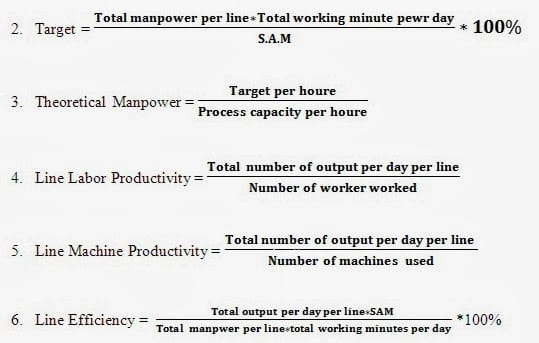Industrial Engineer in RMG Sector
The ready-made garments and exporting industry is facing heavy challenges due to various factors including global competition, increasing production costs, less productivity or efficiency, labor attrition, etc. Now is a time think to about the emerging situation and go deep into the analysis of the situation in a realistic manner. We should upgrade the facilities as system run, rather than people run. The industrial engineering (IE) concept needs to be imparted to the facilities to increase productivity in the garment industry. In this article, I have given some formulas and examples for industrial engineering. I think these formulas and examples will be a handful for an industrial engineer who is doing the job and plans to enter into the industrial engineering (IE) department in the ready-made garment industry.

### Some Essential Formulas and Examples for Industrial Engineer

Standard Pitch Time (S.P.T) = Basic Pitch Time (B.P.T) + Allowances (%)7. GSD = (Man power * Work hour) / Target

8. SMV = Basic time + (Basic time * Allowance)

9. Basic time = Observed time * Rating

10. Observed time = Total Cycle time / No of cycle

11. Rating = (Observed Rating * Standard rating) / Standard rating

12. Efficiency = (Earn minute * Available minute) * 100

13. Earn minute = No of Pc’s (Production) * Garments SMV

14. Available minute = Work hour * Manpower

15. Organization Efficiency = (Basic pis time / Bottle neck time) * 100

16. Basic pis time = Total GMT SMV / Total Manpower

17. UCL = Basic pis time / Organization Efficiency

18. LCL = 2 * Basic pis time – UCL

19. Capacity = 60 / Capacity time in minute

20. Cycle Time = 60 / Team target

21. Capacity Achievable = Capacity * Balance

22. Daily output = Work hour / SMV

23. Factory capacity = (Work hour / SMV) * Total worker * Working day * Efficiency

24. CPM = (Total over head cost of the month / No of SMV earners * Work minutes) * Efficiency

25. Required no of operator = Target daily output / Daily output per operator

Examples:

1. Efficiency = Output/Input

= (SMV * Product Quantity) / (Worker * Working Hour * 60)

= [(38.50 * 700) / (60 * 10 * 60)] * 100

= 74% (Line Efficiency)

Here,
SMV=38.50
Working hour=10
No. of worker=60

2. Target/hour = (60/SMV) * Efficiency%

= (60/0.85)*70%

= 50 pcs/hour

Here,
SMV=0.85
Efficiency=70%

3. Target/line = [(No of worker * working hour * 60)/SMV] * Efficiency%

= [(75 * 10 * 60)/23.5] * 60%

= 1150 pcs

Here,
No of workers=75
Working hours=10
SMV=23.5
Efficiency=60%

4. Required days for the schedule = Total order quantity/Average target

= 50000/2000

=25

Here,
Average target=2000
Order quantity=50000

5. CM of garments= (Total Production) / (no of worker * work hour)

= 250000/(1500*10)

=1.66 BDT

Here,
Total Production=250000
No of workers= 1500

6. Factory capacity = [(Work hour * total workers * working day * 60) / SMV] * Efficiency

= [(10 * 1500 * 26 * 60)/14.13] * 55%

= 828025 pcs/month

7. Line GSD/day = (Manpower * work hour) / target

= (50 * 10 * 60)/900

= 33

If you have any questions, feel free to ask in the comments below. I try my best to respond to every comment that comes my way. If for any reason you don’t get a response, feel free to ask me on Twitter, or Facebook. If you want to follow me on those social media links as well to see different pictures and just talk about different things going on in the tech world.